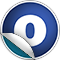scalaz

# Leibniz

#### object Leibniz extends AnyRef

Linear Supertypes
AnyRef, Any
Ordering
1. Alphabetic
2. By inheritance
Inherited
1. Hide All
2. Show all
1. Leibniz
2. AnyRef
3. Any
Visibility
1. Public
2. All

### Type Members

1. #### type === [A, B] = Leibniz[\$u22A5, \$u22A4, A, B]

(A === B) is a supertype of Leibniz[L,H,A,B]

Attributes
sealed

### Value Members

1. #### def != (arg0: AnyRef): Boolean

Attributes
final
Definition Classes
AnyRef
2. #### def != (arg0: Any): Boolean

Attributes
final
Definition Classes
Any
3. #### def ## (): Int

Attributes
final
Definition Classes
AnyRef → Any
4. #### def == (arg0: AnyRef): Boolean

Attributes
final
Definition Classes
AnyRef
5. #### def == (arg0: Any): Boolean

Attributes
final
Definition Classes
Any
6. #### def asInstanceOf [T0] : T0

Attributes
final
Definition Classes
Any
7. #### def clone (): AnyRef

Attributes
protected[lang]
Definition Classes
AnyRef
Annotations
@throws()
8. #### def eq (arg0: AnyRef): Boolean

Attributes
final
Definition Classes
AnyRef
9. #### def equals (arg0: Any): Boolean

Definition Classes
AnyRef → Any
10. #### def finalize (): Unit

Attributes
protected[lang]
Definition Classes
AnyRef
Annotations
@throws()
11. #### def force [L, H >: L, A >: L <: H, B >: L <: H] : Leibniz[L, H, A, B]

Unsafe coercion between types.

Unsafe coercion between types. force abuses asInstanceOf to explicitly coerce types. It is unsafe, but needed where Leibnizian equality isn't sufficient

12. #### def getClass (): java.lang.Class[_]

Attributes
final
Definition Classes
AnyRef → Any
13. #### def hashCode (): Int

Definition Classes
AnyRef → Any
14. #### def isInstanceOf [T0] : Boolean

Attributes
final
Definition Classes
Any

Attributes
implicit
16. #### def lift [LA, LT, HA >: LA, HT >: LT, T[_ >: LA <: HA] >: LT <: HT, A >: LA <: HA, A2 >: LA <: HA] (a: Leibniz[LA, HA, A, A2]): Leibniz[LT, HT, T[A], T[A2]]

We can lift equality into any type constructor

17. #### def lift2 [LA, LB, LT, HA >: LA, HB >: LB, HT >: LT, T[_ >: LA <: HA, _ >: LB <: HB] >: LT <: HT, A >: LA <: HA, A2 >: LA <: HA, B >: LB <: HB, B2 >: LB <: HB] (a: Leibniz[LA, HA, A, A2], b: Leibniz[LB, HB, B, B2]): Leibniz[LT, HT, T[A, B], T[A2, B2]]

We can lift equality into any type constructor

18. #### def lift3 [LA, LB, LC, LT, HA >: LA, HB >: LB, HC >: LC, HT >: LT, T[_ >: LA <: HA, _ >: LB <: HB, _ >: LC <: HC] >: LT <: HT, A >: LA <: HA, A2 >: LA <: HA, B >: LB <: HB, B2 >: LB <: HB, C >: LC <: HC, C2 >: LC <: HC] (a: Leibniz[LA, HA, A, A2], b: Leibniz[LB, HB, B, B2], c: Leibniz[LC, HC, C, C2]): Leibniz[LT, HT, T[A, B, C], T[A2, B2, C2]]

We can lift equality into any type constructor

19. #### def lower [LA, HA >: LA, T[_ >: LA <: HA], A >: LA <: HA, A2 >: LA <: HA] (t: \$eq\$eq\$eq[T[A], T[A2]]): Leibniz[LA, HA, A, A2]

Emir Pasalic's PhD thesis mentions that it is unknown whether or not `((A,B) === (C,D)) => (A === C)` is inhabited.

Emir Pasalic's PhD thesis mentions that it is unknown whether or not `((A,B) === (C,D)) => (A === C)` is inhabited.

Haskell can work around this issue by abusing type families as noted in Leibniz equality can be injective (Oleg Kiselyov, Haskell Cafe Mailing List 2010) but we instead turn to force.

21. #### def ne (arg0: AnyRef): Boolean

Attributes
final
Definition Classes
AnyRef
22. #### def notify (): Unit

Attributes
final
Definition Classes
AnyRef
23. #### def notifyAll (): Unit

Attributes
final
Definition Classes
AnyRef
24. #### implicit def refl [A] : Leibniz[A, A, A, A]

Equality is reflexive -- we rely on subtyping to expand this type

Equality is reflexive -- we rely on subtyping to expand this type

Attributes
implicit
25. #### def symm [L, H >: L, A >: L <: H, B >: L <: H] (f: Leibniz[L, H, A, B]): Leibniz[L, H, B, A]

Equality is symmetric

26. #### def synchronized [T0] (arg0: ⇒ T0): T0

Attributes
final
Definition Classes
AnyRef
27. #### def toString (): String

Definition Classes
AnyRef → Any
28. #### def trans [L, H >: L, A >: L <: H, B >: L <: H, C >: L <: H] (f: Leibniz[L, H, B, C], g: Leibniz[L, H, A, B]): Leibniz[L, H, A, C]

Equality is transitive

29. #### def wait (): Unit

Attributes
final
Definition Classes
AnyRef
Annotations
@throws()
30. #### def wait (arg0: Long, arg1: Int): Unit

Attributes
final
Definition Classes
AnyRef
Annotations
@throws()
31. #### def wait (arg0: Long): Unit

Attributes
final
Definition Classes
AnyRef
Annotations
@throws()
32. #### implicit def witness [A, B] (f: \$eq\$eq\$eq[A, B]): (A) ⇒ B

We can witness equality by using it to convert between types We rely on subtyping to enable this to work for any Leibniz arrow

We can witness equality by using it to convert between types We rely on subtyping to enable this to work for any Leibniz arrow

Attributes
implicit